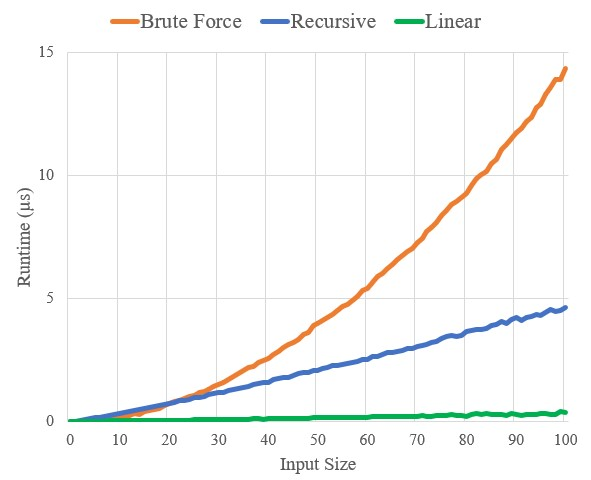Use the following ideas to develop a non-recursive, linear-time algorithm for the maximum-subarray problem. Start at the left end of the array, and progress toward the right, keeping track of the maximum subarray seen so far. Knowing a maximum subarray of $$A[1 \dots j]$$, extend the answer to find a maximum subarray ending at index $$j + 1$$ by using the following observation: a maximum subarray of $$A[1 \dots j + 1]$$ is either a maximum subarray of $$A[1 \dots j]$$ or a subarray $$A[i \dots j + 1]$$, for some $$1 \le i \le j + 1$$. Determine a maximum subarray of the form $$A[i \dots j + 1]$$ in constant time based on knowing a maximum subarray ending at index $$j$$.

The idea is to determine whether $$A[j + 1]$$ will be part of the maximum subarray of $$A[1 \dots j + 1]$$ or not. If it is a part of the maximum subarray, then we will update our maximum subarray to include $$A[j + 1]$$, otherwise we will maintain a temporary sum including $$A[j + 1]$$ in case we encounter a sufficiently large element later which can make the future sum greater than the present sum.

In other words, maintain two sum: a maximum subarray ending at current element, and maximum subarray so far.

$$\textsc {Find-Maximum-Subarray-Linear }(A, low, high)$$\begin{aligned}1& \quad best=-∞ \\2& \quad current=0 \\3& \quad left=0 \\4& \quad right=0 \\5& \quad currentLeft=0 \\6& \quad \textbf {for }i=low\textbf { to }high \\7& \quad \qquad current=current+A[i] \\8& \quad \qquad \textbf {if }current>best \\9& \quad \qquad \qquad best=current \\10& \quad \qquad \qquad left=currentLeft \\11& \quad \qquad \qquad right=i \\12& \quad \qquad \textbf {if }current<0 \\13& \quad \qquad \qquad current=0 \\14& \quad \qquad \qquad currentLeft=i+1 \\15& \quad \textbf {return }\textsc {}(left,\,right,\,best) \\\end{aligned}

In line 7, we update our current sum involving current element. And in lines 8-11, we update our best subarray, if required. In lines 12-14, we reset current running sum if it is negative.

A python implementation of this pseudocode is available at the bottom of the page.

#### Runtime Comparison

We can measure the performance of this algorithm along with others as described in Exercise 4.1.3. As expected the linear algorithm runs much much faster than other algorithms (running times are in microseconds):

Size BruteForce Recursive Linear
20 1.1168 1.2608 0.2176
21 1.1319 1.3312 0.2278
22 1.2343 1.3643 0.2445
23 1.3397 1.4459 0.2512
24 1.4235 1.5344 0.2537
25 1.5350 1.6118 0.2664
26 1.6584 1.6801 0.2766
27 1.7502 1.7495 0.2921
28 1.8662 1.8276 0.3040
29 1.9923 1.8878 0.3043
30 2.1180 1.9716 0.3153
31 2.2915 2.0271 0.3507

The C++ implementation to generate this data can be downloaded from here.

And here is a visual representation of the runtime comparison.As you can see the brute force run time, $$\Theta(n^2)$$, very closely resembles a quadratic curve. Runtime of recursive method is $$\Theta(n \lg n)$$ and for linear it is $$\Theta(n)$$, the difference between them also can be seen clearly, although both resembles linear curve. That ambiguity will reduce if we can create graph for much higher input size, e.g. $$n = 1000$$.

#### Python Implementation

import math def FindMaxSubarrayLinear(A, low, high): best = -math.inf current = 0 left = 0 right = 0 current_left = 0 for i in range(low, high): current += A[i] if current > best: # Update best sum best = current left = current_left right = i if current < 0: # Reset current sum current = 0 current_left = i + 1 return (left, right, best) # Test with exact same array as in the example give in book # Expected answer is A[8..11] = 43 arr = [13, -3, -25, 20, -3, -16, -23, 18, 20, -7, 12, -5, -22, 15, -4, 7] ans = FindMaxSubarrayLinear(arr, 0, len(arr)) print(f"Max subarray = A[{ans + 1}..{ans + 1}] = {ans}")